Your browser doesn't support the features required by impress.js, so you are presented with a simplified version of this presentation.

For the best experience please use the latest Chrome, Safari or Firefox browser.

FOSDEM'17
The power of duck typing and linear algrebra

Track: Python devroom
Room: H.1308 (Rolin)
Day: Saturday
Start: 11:30
End: 12:00

(me too, I love to have the slides during presentation so here is the url http://jul.github.io/cv/pres.html)
All great story begins with a book! And too much free time

# Part1: The magic of algebra

(and so is duck typing)
Legal discolusure: My linear algebra books is the french version of
Lipschutz Linear Algebra (Shaum edition)

# Linear algebra 101:

If you behave nicely and consistently you have super cow powers including

• metrics
• distributive systems, paralellism
• least surprises

# Theorem 1: given simple enough rules you can make TDD

Linear algebra definition is basically if you pass the tests, then you have super powers.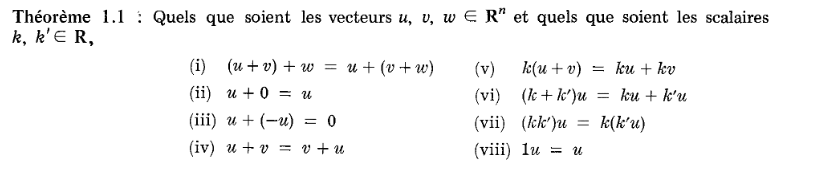Nota Bene:
• 1 is NOT 1, and so 0 is not 0. They are symbol that does the job
• Computer int are no integer, floats are not real numbers, and all is lies
• Good enough lies worth a good enough truth

# Step 0: Writing you own test frameworks

Doesn't it look like the exact perfect match case of a unit test?
Consistent Algebra test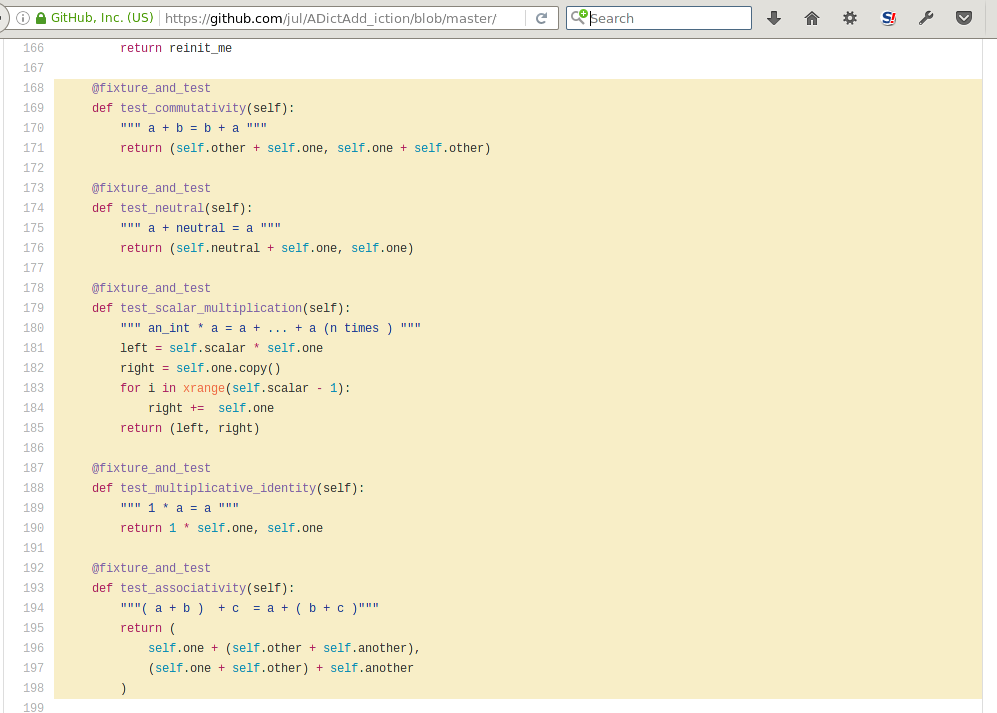# Which makes you curious of what is compliant by default

(yes I purposefully did not went on edge cases)
**************************************************
testing for  'complex' class
a = (1+0j)
b = (1+1j)
c = (1-4j)
an_int = 2
other_scalar = 3
neutral element for addition is 0j

**************************************************

test #1
a + b = b + a
'test_commutativity' is 'ok'

test #2
a + neutral = a
'test_neutral' is 'ok'

... other tests ....

test #12
an_int ( a + b) = an_int *a + an_int * b
'test_distributivity' is 'ok'

**************************************************

14/14
<type 'complex'> respects the linear algebrae standard rules

**************************************************


# Just for fun what default type can be considered scalars?

Type Neutral of add. Neutral of mul. Result
Numpy array zeroes(dim) ones(dim) OK
floats 0.0 1.0 OK
int 0 1 OK
Decimal Decimal(0.000000) Decimal(1.000000) KO
complex 0+0j 1+0j OK
list [ ] 1 KO
You can also test strings, quaternions....

Yes, math rox, you are free to code whatever you want and you don't have to justify youself, as long as you pass the tests

Note: it has nothing to do with coconut/rust algebraic data type as explained on wikipedia. I would say, if I would understand it.

# Let's make a simple observation

On a MutableMapping anything that is not a leaf is a part of a key.
But let's turn
{ 'a': { 'b' : 1 }, 'b' : 2} AS
 { ('a', 'b') : 1; 'b': 2 }
All keys are immutable. So the tuple of the keys is also...immutable.
Hence:

Every distinct immutables path to a value are a key, and then we can reduce a recursive problem to a 1 level problem.
All MutableMapping are utimately possibly put in a canonical one level form.
Every distinct immutable paths to a value are equivalent to a dimension.
We have an isomorphism between the one level MutableMapping and arbitrary depth MutableMappings.

We transformed a recursive/deep level problem in a one depth problem.

# Math books then gives you the Cook book

(did I pronounced meth books?)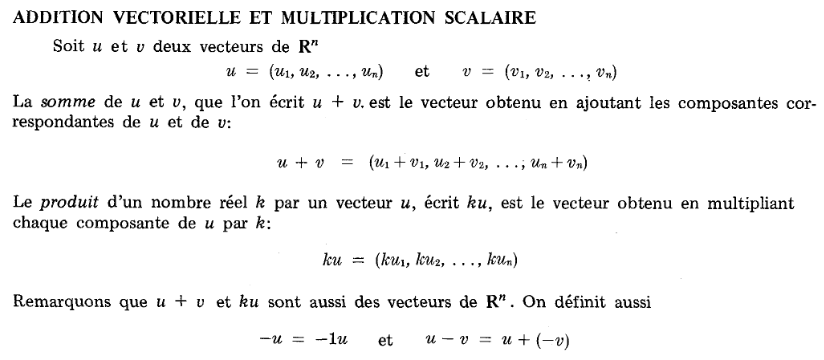# Part 2: making dict follow linear algebra behaviour

Arbitrary choices:

• - (___sub__, __rsub__, __isub__)
• * (___mul__, __rmul__, __imul__)
• / (___div__, __rdiv__, __idiv__)
and if a left and right are mutable mapping aka dict, I propagate as long as both left/right operantd match key path to a leaf
• I made junction of relationships such as defining __neg__ (unary -) as
__neg__ = lambda self: self*=-1
to ensure consistency
Abstract base class* (type) duck typing and operator overloading are the only 2/3 tricks used.

The exact same trick can probably be implemented in C++, in ruby, Perl, PHP, javascript...

# Part 3: And? Does it pass the tests?

At least for the simple case...
**************************************************

testing for  'Daikyu' class

a = {'one_and_two': 3, 'one': 1}
b = {'two': 2, 'one_and_two': -1}
c = {'one': 3, 'three': 1, 'two': 2}
an_int = 2
other_scalar = 3
neutral element for addition is {}

**************************************************
... 16 tests later ...
**************************************************

16/16
respects the linear algebrae standard rules

**************************************************

Yes!!!

We have a one level dict behaving as a vector...

# Part 4: Let's use the isomorphism to make intricate dict into vector let's implement, cos, dot

from collections import MutableMapping

MARKER=object()

def paired_row_iter(tree, path=MARKER):
if path is MARKER:
path = tuple()

for k, v in tree.iteritems():
if isinstance(v, MutableMapping) and len(v):
for child in paired_row_iter(v, path + (k,)):
yield child
else:
yield ((path + (k,)) , v)

dict(paired_row_iter({ 'a' : { 'b' :1},  'c':2, 'd' : {'e': []}, 'e': {} }) )
# {('a', 'b'): 1, ('c',): 2, ('d', 'e'): [], ('e',): {}}



# let's read the specs of the dot product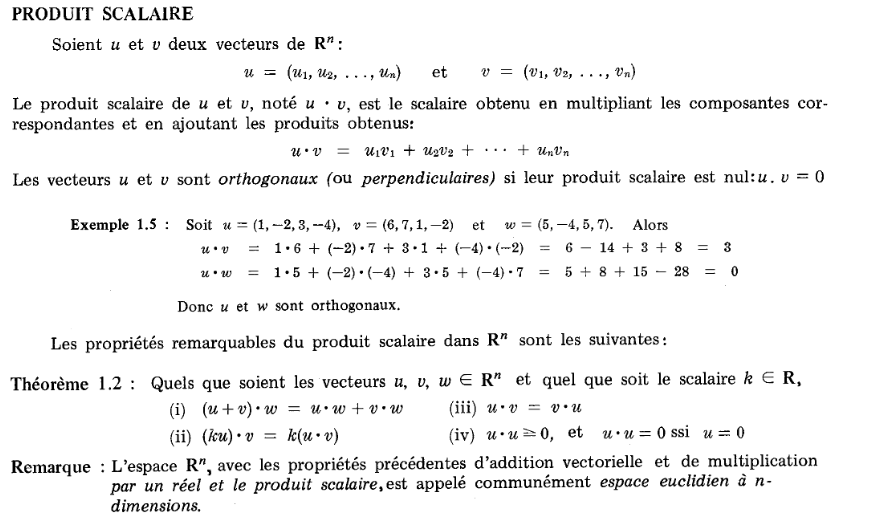# Let's implelement them

NB: Purists want measure u.u to be
• defined
• normed
• positive
to fit with the Lebesgue measure.
My distance however does yield a complex, if scalars are complex.
dot = lambda u,v: sum(v for i,v in paired_row_iter(u*v))

dot(mdict(x=1,y=1, z=1), mdict(x=1, y=1, z=1))
# 3
dot(mdict(x=1,), mdict( y=1, z=1))
# 0
dot(mdict(x=1,), mdict(x=-1, y=1, z=1))
# -1

For int and real, the resutls are following expectation.
For complex, I am pretty clueless if it is normal.

# Please stop the roto-zoom slides

Yes I noticed the persons on the last row being sea sick.
Social experiment #154 over: roto-zoom presentation make people uncomfortable. Lol

# Dot product is one step away from distance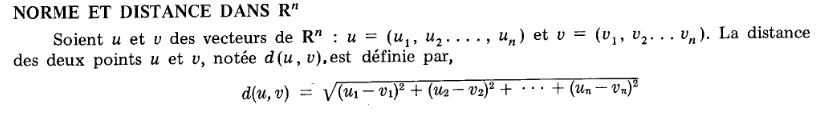Now we can define abs (which is a mathemtic shortcut for L2 distance/norm) and distance as
vabs = lambda v: dot(v,v)**.5
distance = lambda u, v: vabs(u-v)

It would be more aesthetic for vector of dimension p to write. $$\left\| x \right\| _p = \bigg( \sum_{ i \in \mathbb N} \left| x_i \right| ^p \bigg) ^{1/p}$$
However L2 norm, is making coding way easier, so I totally agree.

# And not far from the ... cosinus

(math pun are a sine)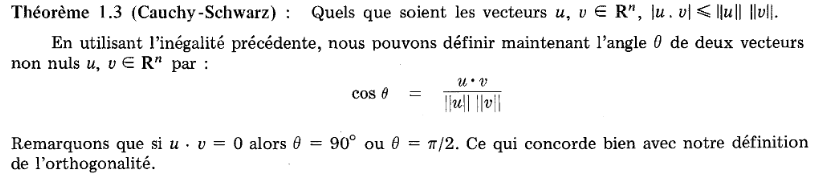vcos = lambda u,v : dot(u,v) / vabs(u) / vabs(v)

vcos(mdict(x=1, y=1), mdict(x=1, y=0))
# Out: 0.7071067811865475

2**.5/2
# Out: 0.7071067811865476 (cos(45°)

vcos(mdict(x=1, ), mdict(x=2, y=4, z=4, v=4))
# Out: 0.3333333333333333  (cos 60°)

vcos(mdict(x=1), mdict())
# ZeroDivisionError: float division by zero

So far so good.

We have something that is built for infinite intricated dimensions, and that already works for the 2D & 3D euclidean space without even trying.
And we even have a meaningful exception: cos with a null vector does not mean a thing.

Gosh :) Sorry

# But does it have the promised behaviour?

Let's check.
Can we mathematically expose an API that following the least surprise principle:
• for computers? (checked)
• for coders? (it works for me)
• actual mathematical use? (...)
Especially, is it useful?
intuitive?
working correctly?

# Let's watch the behaviour of more complex data

Time to switch to ipython notebook \o/

# Use case?

• Text indexation
• aggregating log parsing (cf yahi on github).
Or simply pypi-stats
Better known as pypi-ranking (for which I am not credited, bouhouh)
• dict manipulations and measurement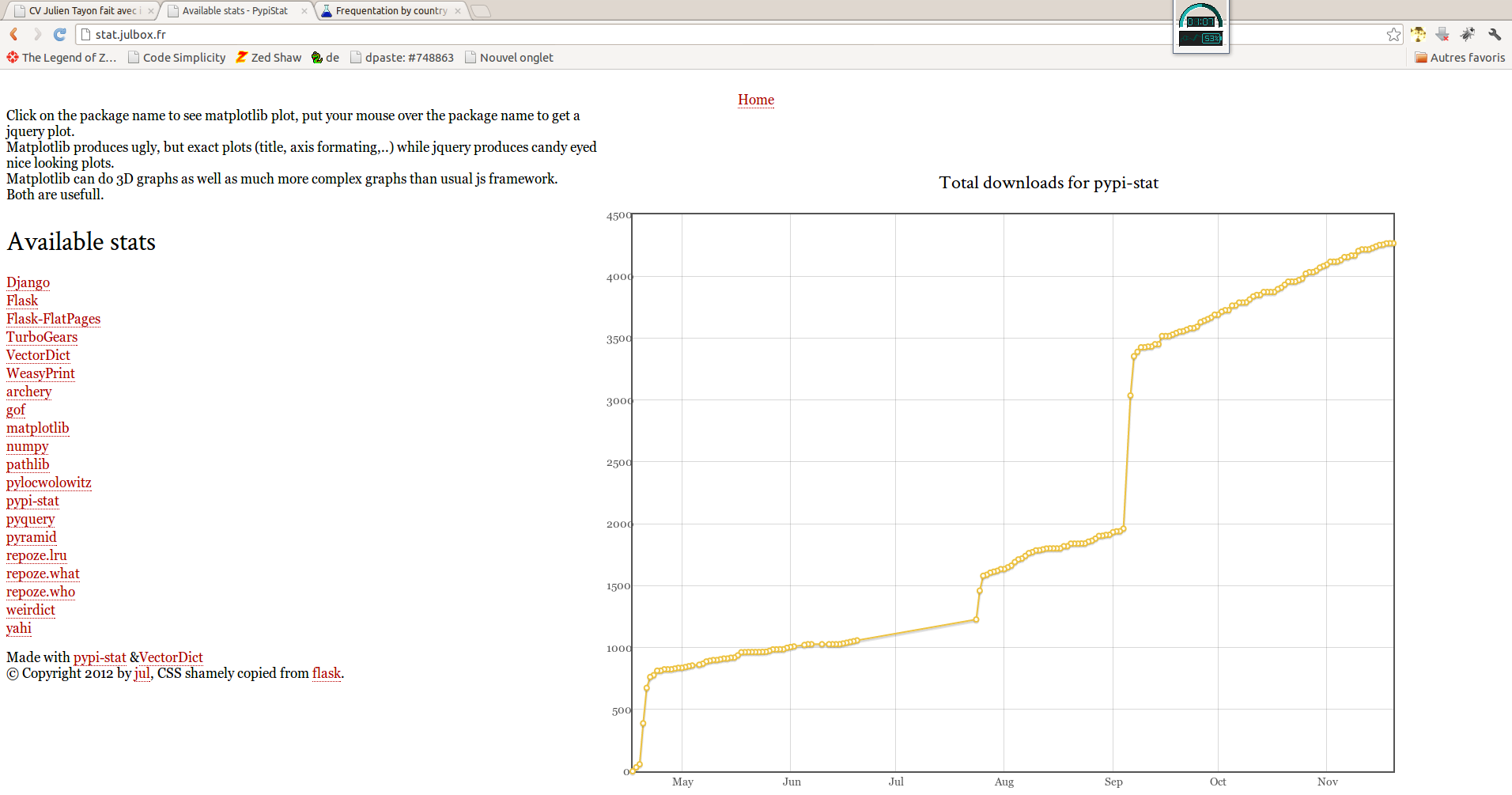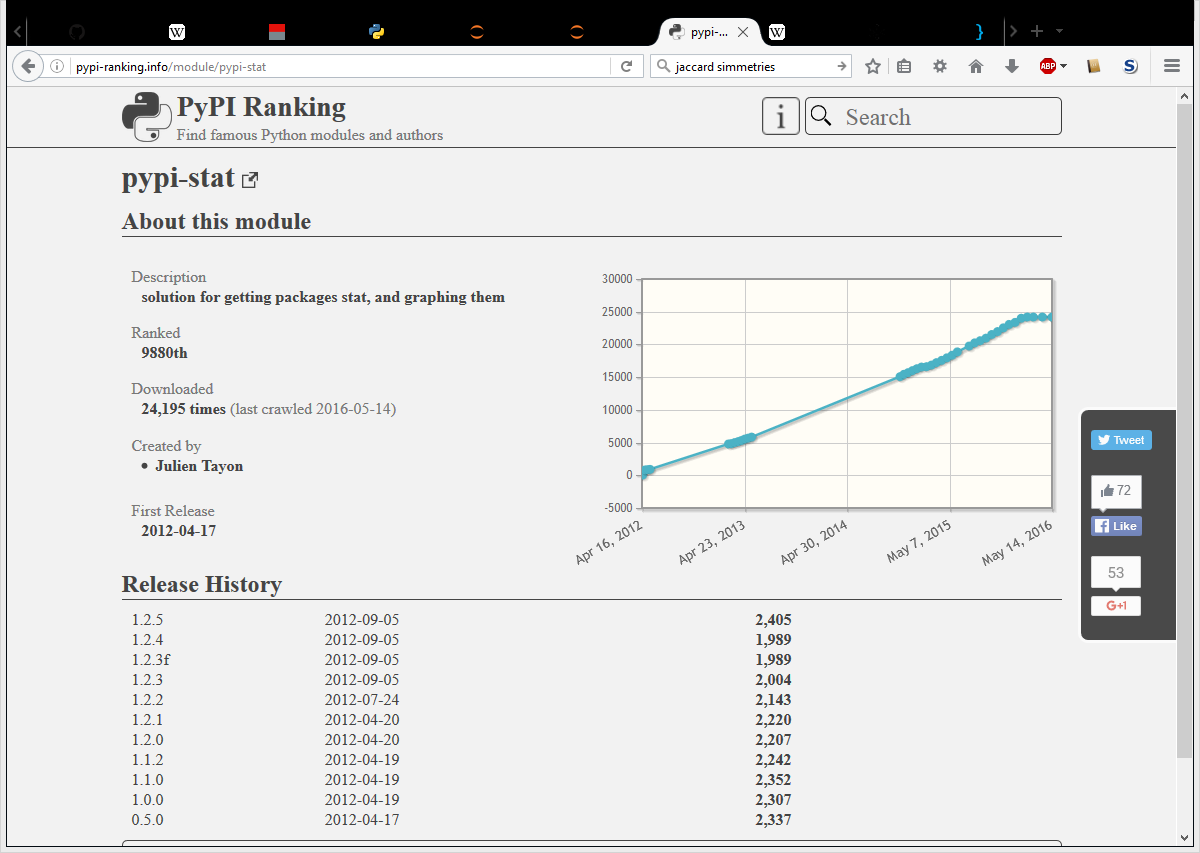# An orthogonal rant on OOP introduction with the 2D point class

(oh! a geometrical joke)
Complex type does EVERYTHING better than any 2D class I saw!
Maths are powerful, use them: check here
python3 documentation has reduced considerably the Point example in the collections doc
I cannot say how much I consider the 2D class Point as misleading as Hello world

# Parellism you said?

(Another geometrical joke)

Our boring data job? Take a dict (like after we parse a log line, or cvs) and aggregating the data in a dict.

log_line = mdict( ip = "127.0.0.1", user_agent = "moz", country="BE",
url="/toto" )
data = mdict( by_country = mdict( GR=12, CA=10), user_agent=mdidct(chrome=1,  ie=1000,))
data["user_agent"] += log_line * mdict( user_agent = 1)


What if we don't have a dict, but just a MutableMapping that directs __getitem__ and __setitem__ to a distant instance?

# Should I use your library?

• migration to py3 stopped since 3 years (unexpected events occured)
• It works for me(c)
• the naming is awfull and what was indentend as puns is a pain
• for dimension greater than 3 results are consistent
• I did it alone, there is no great mind that can grant this is correct
• Some bugs corrected during presentation not yet pushed to archery (v0.2)
VectorDict is used O_o (I still have to push a pull request in pypi). I DO NOT recommend it, except for seeing the features I was initially encompassing.
Archery implementing the mixin separately at my opinion is quite mature. BUT, I have trust issues, because I lack of feedback.
I hate the naming and how I pedantically javafied the module layout.
I strongly advise to see this code as an academic work and a proof of concept that is delivered AS IS

# And have you tried proposing to python-ideas?

Lol
I tried.
Not a great communicator# Thanks to:

• Stéphane Wirtel, and FOSDEM team
• Pierre Parisot (who taught me everything @IBM ECAM) on text indexation,
• YOU!All the great coders that make FOSS fun!
• @bartaz the author of impressjs used for this pres
• Mc Graw Hill edition without which I would never have access to cheap non pedantic books during my studies (okay, I should also include O'Reilly)

Use a spacebar or arrow keys to navigate